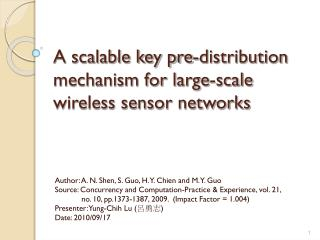Download PresentationA scalable key pre-distribution mechanism for large-scale wireless sensor networks

# A scalable key pre-distribution mechanism for large-scale wireless sensor networks

Download Presentation## A scalable key pre-distribution mechanism for large-scale wireless sensor networks

- - - - - - - - - - - - - - - - - - - - - - - - - - - E N D - - - - - - - - - - - - - - - - - - - - - - - - - - -
##### Presentation Transcript

1. A scalable key pre-distribution mechanism for large-scale wireless sensor networks Author: A. N. Shen, S. Guo, H. Y. Chien and M. Y. Guo Source: Concurrency and Computation-Practice & Experience, vol. 21, no. 10, pp.1373-1387, 2009. (Impact Factor = 1.004) Presenter: Yung-Chih Lu (呂勇志) Date: 2010/09/17

2. Outline • Introduction • Improved Key Distribution Mechanism • Proposed Scheme • Performance Evaluation • Security Analysis • Conclusion • Comment

3. Introduction (1/4) • Large-scale WSN WSN: Wireless Sensor Network

4. Introduction (2/4) • Goal • Key agreement • Against attack • node capture attack • Saving resource • communication cost • computation cost • storage overhead

5. [] 5　０　０　０ ０　０　０　１ ０　０　４　０ ０　１　０　０ Introduction (3/4) C. Blundo, A.D. Santis, A. Herzberg, S. Kutten, U. Vaccaro, M. Yung. "Perfectly-secure Key Distribution for Dynamic Conferences." Lecture Notes in Computer Science,471–486 , 1993. • Blundo Polynomial-based protocol • Setup server randomly generates a symmetric bivariate t-degree polynomial Example: f(x,y) = 4x2y2 + x3y1+ x1y3 + 5 It’s a symmetric bivariate 3-degree polynomial

6. Introduction (4/4) • Blundo Polynomial-based protocol step1: computes 1: Cluster Head ID 2: Lv-sensor ID f(1,y) = 4y2 + y1 + y3 + 5 f(2,y) = 16y2 + 8y1 + 2y3 + 5 step2: The Setup server loads the sensor node with coefficients step3: Each sensor node broadcasts its own ID step4: Receiver use ID to compute a shared secret key Kuv = f(u,v) = f(v,u) = Kvu K12 = f(1,2) = 31 = f(2,1) = K21 :Cluster head y0 y1 y2 y3 s4 s3 s4 :Lv-sensor y0 y1 y2 y3 L-sensor: Low-end sensor s: Step

7. Improved Key Distribution Mechanism (1/3) Y. Cheng and D. P. Agrawal, "improved key distribution mechanism for large-scale hierarchical wireless sensor networks." Journal of Ad Hoc Networks, vol.5, no.1, pp.35–48, 2007. • Key pre-distribution phase • Cluster head • Sensor node • CHa id and CHb id BS: base station f(x,y): t-degree bivariate symmetric polynomial CHi: Cluster head i CH: Set of cluster heads in a network Si: Sensor node i KA-B: Symmetric key between A and B

8. Improved Key Distribution Mechanism (2/3) • Inter-cluster pairwise key establishment • step1: CHa and CHb exchange their node id each other • step2: :CHa s2 s1 s2 :CHb f(x,y): t-degree bivariate symmetric polynomial CHi: Cluster head i CH: Set of cluster heads in a network KA-B: Symmetric key between A and B s: step 8

9. Improved Key Distribution Mechanism (3/3) • Intra-cluster pairwise key establishment • step1: Si sends its id, CHa id and CHb id to its CHj. • step2: CHj sends Si to CHa and CHb. • step3: CHu sends back • to CHj. • , t=1,2 u=a,b • step4: CHj decrypts • to get kt. • step5: CHa: s3 s2 s2 s4,s5 :CHj s3 :CHb s1 :Si CHj: Cluster head j Si: Sensor node I s: step

10. Proposed Scheme (1/3) • Key pre-assignment phase • Cluster head • Sensor node BS: base station KA,B: Symmetric key between A and B CHi: Cluster head i with IdSNi: Sensor node i with Id f(x,y): t-degree bivariate symmetric polynomial

11. Proposed Scheme (2/3) • Inter-cluster pair-wise key establishment phase s3,s4 :CHa s1,s2 Step1: s3,s4 :CHb Step2: Step3: Step4:

12. Proposed Scheme (3/3) • Intra-cluster pair-wise key establishment phase Step1: Step2: Step3: Step4:

13. Performance Evaluation (1/2) • Storage cost and Computational cost n: the number of low-end sensor node t: the degree of polynomial m: the number of cluster head SKPD: our scheme

14. Performance Evaluation (2/2) • Communication cost LEKM: Low-Energy Key Management SKPD: our scheme IKDM: Improved Key Distribution Mechanism

15. Security Analysis (1/2) • Initialization of the network LEKM: Low-Energy Key Management SKPD: our scheme IKDM: Improved Key Distribution Mechanism

16. Security Analysis (2/2) • After deployment of the network LEKM: Low-Energy Key Management SKPD: our scheme IKDM: Improved Key Distribution Mechanism

17. Conclusion • Best network resilience against node capture attack • Communication overhead scheme is zero • lowest energy consumption • Reduces the key storage overhead • Suitable for large-scale WSNs

18. Comment • elasticity of demand is a lack of this scheme. • other attacks